Looking for VBA Tutorials?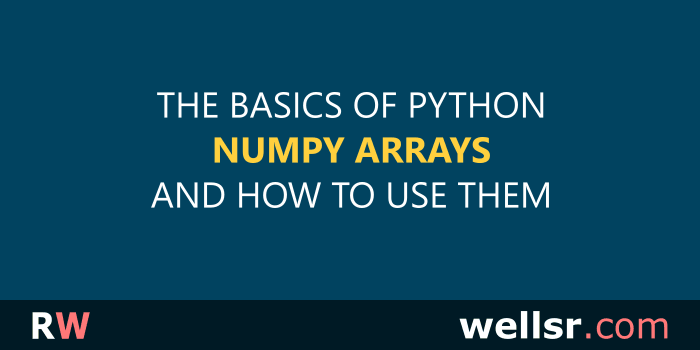#### Nov 27, 2019

This tutorial introduces the Python NumPy Library and explains how to use it to create arrays and perform arithmetic and matrix operations on NumPy arrays.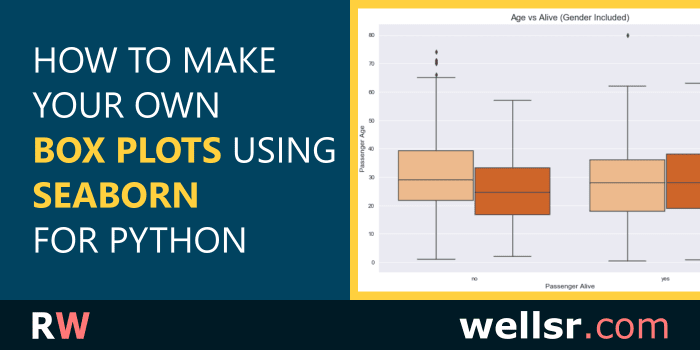#### Nov 22, 2019

This tutorial draws different Seaborn Boxplots using the Python Seaborn library. It includes examples for editing the colors, columns and labels of a box plot.#### \$120.00 \$57.00

Want to learn more programming languages? We've combined each of our comprehensive VBA reference guides into a single bundle with over 200 tips and macros covering the 125 most important topics in VBA.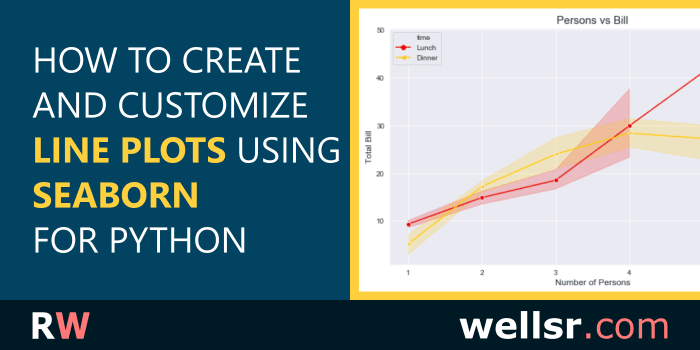#### Nov 12, 2019

This tutorial explains how to draw different line plots using the Python Seaborn library. It includes examples for setting line plot labels, markers and more.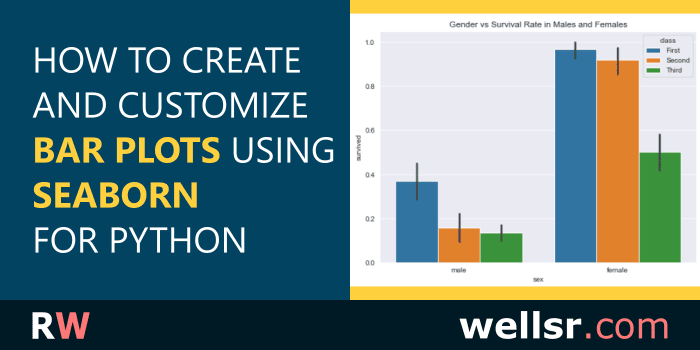#### Oct 25, 2019

This tutorial explains how to use the Seaborn barplot function in Python, including how to make grouped bar plots, bar plots with values and barplot titles.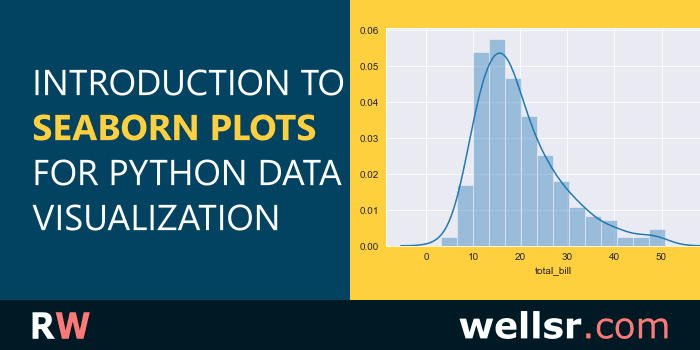#### Oct 18, 2019

This tutorial introduces the Python Seaborn library for data visualization and includes Seaborn plot examples so you can see how it helps visualize Python data.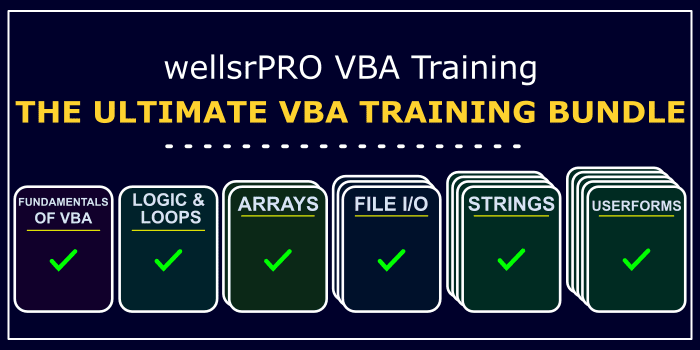#### \$120.00 \$57.00

We created a suite of 6 VBA cheat sheets with over 200 tips showing you everything you need to know to start making power Excel applications. Take a look!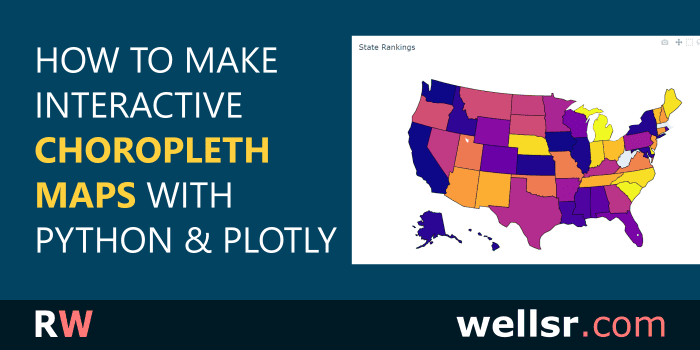#### Sep 7, 2019

This tutorial will show you how to create an interactive choropleth map of US States using Python and Plotly. A choropleth map is a geographic color heatmap.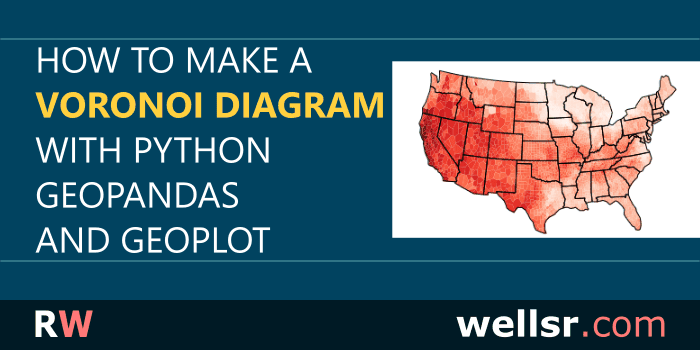#### Aug 23, 2019

This tutorial will teach you how to create a Python Voronoi Diagram over a map of the United States using a Pandas DataFrame with GeoPandas and Geoplot.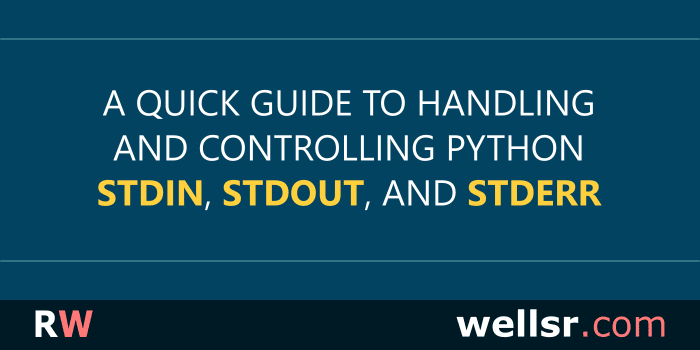#### Aug 9, 2019

This tutorial will explain how to use shell standard input (stdin), standard output (stdout), and standard error (stderr) in Python programs using the sys module.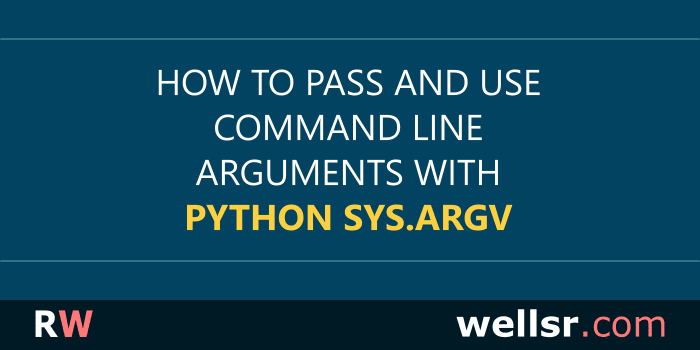#### Jul 26, 2019

This tutorial describes how to use command line arguments passed to a Python script execution with the sys module's argv list.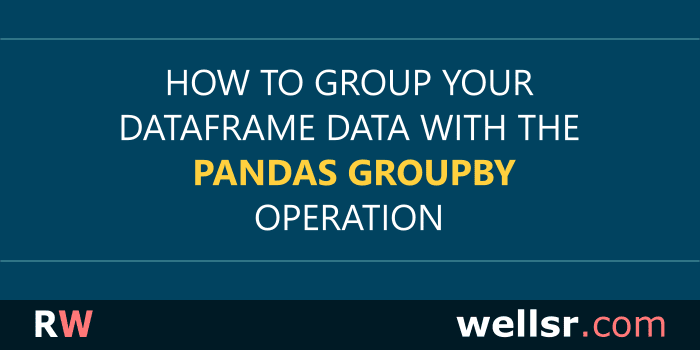#### Jul 12, 2019

Use the Python Pandas groupby operation to group and aggregate data in a DataFrame. The Pandas groupby operation can group data by a single or multiple columns.